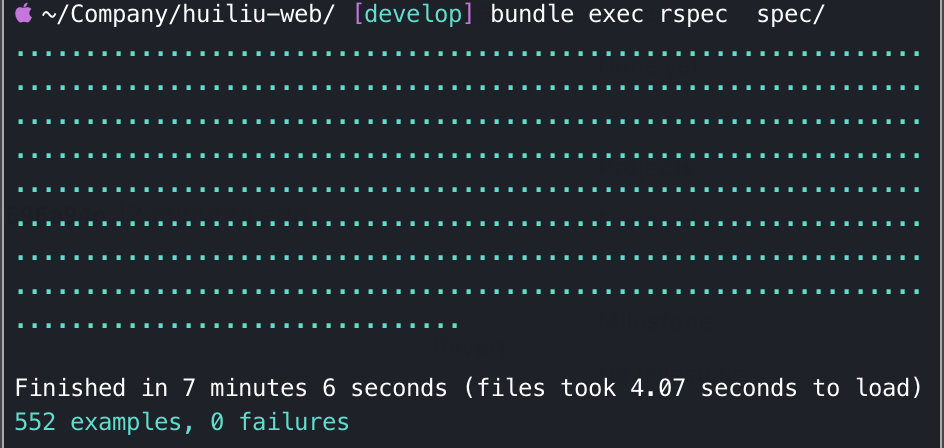# 前言# 如何写测试

## 1. 自顶向下

``````（用代码的方式）打开浏览器 --> 在浏览器输入接口的URL --> 点击确认 --> 等待页面加载 --> 检查页面内容（或者返回的数据）是否符合预期
``````

## 2. 自底向上

``````class A
def end_method
return_a + return_b + return_c + return_d
end

private

def return_a
'a'
end

def return_b
'b'
end

def return_c
'c'
end

def return_d
'd'
end
end
``````

``````RSpec.describe do
describe 'method' do
let(:instance) { A.new }

it 'test size private methods' do
expect(instance.send(:return_a)).to eq('a')
expect(instance.send(:return_b)).to eq('b')
expect(instance.send(:return_c)).to eq('c')
expect(instance.send(:return_d)).to eq('d')
end

it 'test public method' do
expect(instance.end_method).to eq('abcd')
end
end
end
``````

``````RSpec.describe do
describe 'method' do
let(:instance) { A.new }

it 'test public method' do
expect(instance.end_method).to eq('abcd')
end
end
end
``````

# 测试策略

1. 日常运营所需的后台管理系统。
2. 小程序应用所需要的接口。

## 1. 有选择地去测试

``````def return_true
true
end

RSpec.describe do
it 'test true' do
expect(return_true).to be(true)
end
end
``````

## 2. 错误导向测试

• 前端跟我说接口漏了几个字段？马上编写测试保证接口会返回相应的字段。
• 接口接收参数的时候没有做空判断，导致传入空值的时候会报500的异常？立马编写参数为空值的测试用例，然后修改代码让这个测试通过。
• 数据库出现了跟预期不一致的数据？在测试中构造这种奇葩的数据，尝试入库，预期入库失败。然后调整校验代码，让测试通过。

## 3. 尽可能少针对页面布局写测试

``````RSpec.describe do
it do
expect('<p class="qwe rty" id="qwerty">Paragraph</p>').to have_tag('p', :with => { :class => 'qwe rty' })
expect('<p class="qwe rty" id="qwerty">Paragraph</p>').to have_tag('p', :with => { :class => 'rty qwe' })
expect('<p class="qwe rty" id="qwerty">Paragraph</p>').to have_tag('p', :with => { :class => ['rty', 'qwe'] })
expect('<p class="qwe rty" id="qwerty">Paragraph</p>').to have_tag('p', :with => { :class => ['qwe', 'rty'] })
end
end
``````

## 4. 给所有接口都加上测试用例

1. 组装请求链接。
2. 利用客户端发送相关的请求。
3. 检查返回的状态码，如果有必要再检查返回的数据正确性。
4. 检查请求所造成的副作用。

``````describe 'Blogs API' do
path '/blogs/{id}' do
get 'Retrieves a blog' do
tags 'Blogs'
produces 'application/json'
parameter name: :id, in: :path, type: :string

response '200', 'blog found' do
let(:id) { Blog.create(title: 'foo', content: 'bar').id }
run_test! do |response|
# Check the field of result
end
end

let(:id) { 'fake-id' }
run_test!
end
end
end
end
``````

# 推荐技术

## 2. 测试框架

1. 组装api
2. 发送请求
3. 检测响应状态码，还有响应体。